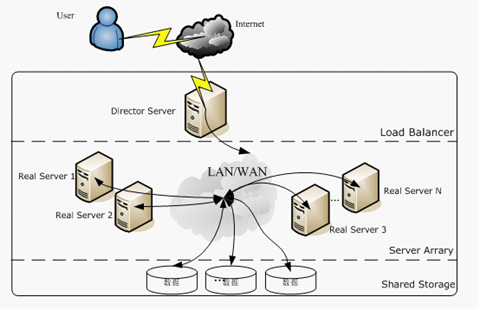数据可视化之核密度估计

Seaborn是一种基于matplotlib的图形可视化python libraty。它提供了一种高度交互式界面，便于用户能够做出各种有吸引力的统计图表。Seaborn其实是在matplotlib的基础上进行了更高级的API封装，从而使得作图更加容易，在大多数情况下使用seaborn就能做出很具有吸引力的图，而使用matplotlib就能制作具有更多特色的图。应该把Seaborn视为matplotlib的补充，而不是替代物。同时它能高度兼容numpy与pandas数据结构以及scipy与statsmodels等统计模式。掌握seaborn能很大程度帮助我们更高效的观察数据与图表，并且更加深入了解它们。但应强调的是，应该把Seaborn视为matplotlib的补充，而不是替代物。

seaborn.kdeplot()

seaborn.kdeplot(data, data2=None, shade=False, vertical=False, kernel='gau', bw='scott', gridsize=100, cut=3, clip=None, legend=True, cumulative=False, shade_lowest=True, cbar=False, cbar_ax=None, cbar_kws=None, ax=None, **kwargs)

• data：一维阵列
• data2：一维阵列，可选。第二输入数据。如果存在，将估计双变量KDE。
• vertical：布尔值，可选参数。如果为True，密度图将显示在x轴。
• kernel：{‘gau’|‘cos’|‘biw’|‘epa’|‘tri’|‘triw }，可选参数。要拟合的核的形状代码，双变量KDE只能使用高斯核。
• bw：{‘scott’ | ‘silverman’ | scalar | pair of scalars }，可选参数。用于确定双变量图的每个维的核大小、标量因子或标量的参考方法的名称。需要注意的是底层的计算库对此参数有不同的交互：statsmodels直接使用它，而scipy将其视为数据标准差的缩放因子。
• gridsize：整型数据，可选参数。评估网格中的离散点数。
• cut：标量，可选参数。绘制估计值以从极端数据点切割。
• clip：一对标量，可选参数。用于拟合KDE图的数据点的上下限值。可以为双变量图提供一对（上，下）边界。
• legend：布尔值，可选参数。如果为True，为绘制的图像添加图例或者标记坐标轴。
• cumulative：布尔值，可选参数。如果为True，则绘制kde估计图的累积分布。
• cbar：布尔值，可选参数。如果为True并绘制双变量KDE图，为绘制的图像添加颜色条。
• cbar_ax：matplotlib axes，可选参数。用于绘制颜色条的坐标轴，若为空，就在主轴绘制颜色条。
• cbar_kws：字典，可选参数。colorbar()的关键字参数。
• ax：matplotlib axes，可选参数。要绘图的坐标轴，若为空，则使用当前轴。
• **kwargs：键值对。其他传递给plot()或plt.contour {f}的关键字参数，具体取决于是绘制单变量还是双变量图。

seaborn.rugplot()

rugplot的功能非常朴素，用于绘制出一维数组中数据点实际的分布位置情况，即不添加任何数学意义上的拟合，单纯的将记录值在坐标轴上表现出来，相对于kdeplot，其可以展示原始的数据离散分布情况。

seaborn.rugplot(a, height=0.05, axis='x', ax=None, **kwargs)

• a：1维的观察数组。
• height：标量，可选。以比例形式表示的坐标轴上棒状标识的高度。
• axis：{‘x’ | ‘y’}, 可选。需要画rugplot的坐标轴
• ax：matplotlib 轴，可选。进行绘制的坐标轴; 未指定的话设定为当前轴。
• kwargs：键值对。被传递给LineCollection的其他关键字参数。

seaborn.distplot()

seaborn的displot()函数结合了matplotlib中的 hist函数、seaborn的kdeplot()和rugplot()函数。它还可以拟合scipy.stats分布并在数据上绘制估计的PDF（概率分布函数）。

seaborn.distplot(a, bins=None, hist=True, kde=True, rug=False, fit=None, hist_kws=None, kde_kws=None, rug_kws=None, fit_kws=None, color=None, vertical=False, norm_hist=False, axlabel=None, label=None, ax=None)

• a：Series、1维数组或者列表。如果是具有name属性的Series对象，则该名称将用于标记数据轴。
• bins：matplotlib hist()的参数，或None。可选参数。直方图bins（柱）的数目，若填None，则默认使用Freedman-Diaconis规则指定柱的数目。
• hist：布尔值，可选参数。是否绘制（标准化）直方图。
• kde：布尔值，可选参数。是否绘制高斯核密度估计图。
• rug：布尔值，可选参数。是否在横轴上绘制观测值竖线。
• fit：随机变量对象，可选参数。一个带有fit方法的对象，返回一个元组，该元组可以传递给pdf方法一个位置参数，该位置参数遵循一个值的网格用于评估pdf。
• hist_kws：底层绘图函数的关键字参数
• kde_kws：底层绘图函数的关键字参数
• rug_kws：底层绘图函数的关键字参数
• fit_kws：底层绘图函数的关键字参数
• color：matplotlib color，可选参数。可以绘制除了拟合曲线之外所有内容的颜色。
• vertical：布尔值，可选参数。如果为True，则观测值在y轴显示。
• norm_hist：布尔值，可选参数。如果为True，则直方图的高度显示密度而不是计数。如果绘制KDE图或拟合密度，则默认为True。
• axlabel：字符串，False或者None，可选参数。横轴的名称。如果为None，将尝试从name获取它；如果为False，则不设置标签。
• label：字符串，可选参数。图形相关组成部分的图例标签。
• ax：matplotlib axis，可选参数。若提供该参数，则在参数设定的轴上绘图。返回值：ax：matplotlib Axes返回Axes对象以及用于进一步调整的绘图。

Freedman-Diaconis rule

$$h = 2\frac{\text{IQR}(x)}{\sqrt{n}}$$

$$k = \lceil \frac{\max{x} – \min{x}} {h}\rceil$$

Seaborn使用实战

%matplotlib inline
import numpy as np
import seaborn as sns
sns.set()

np.random.seed(1024)
x = np.random.randn(100)

sns.kdeplot(x)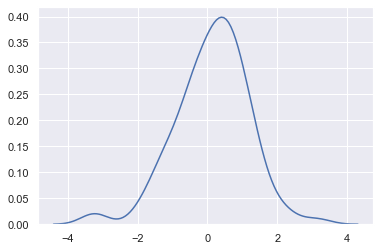cut：参数表示绘制的时候，切除带宽往数轴极限数值的多少(默认为3)

sns.kdeplot(x, cut=0)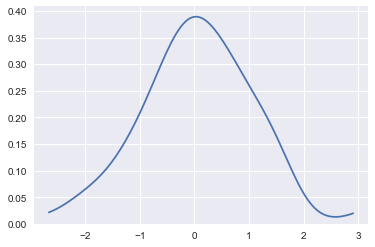cumulative ：是否绘制累积分布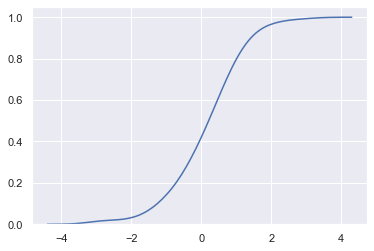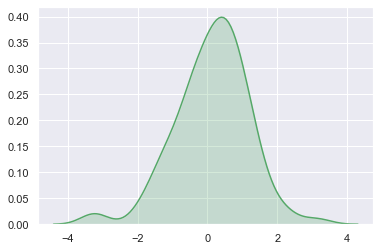vertical：表示以X轴进行绘制还是以Y轴进行绘制

sns.kdeplot(x,vertical=True)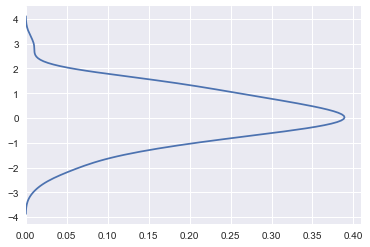KDE的带宽bandwidth（bw）参数控制估计对数据的拟合程度，与直方图中的bin(数据切分数量参数)大小非常相似。 它对应于我们上面绘制的内核的宽度。 默认中会尝试使用通用引用规则猜测一个适合的值，但尝试更大或更小的值可能会有所帮助：

sns.kdeplot(x)
sns.kdeplot(x, bw=.2, label="bw: 0.2")
sns.kdeplot(x, bw=2, label="bw: 2")
plt.legend();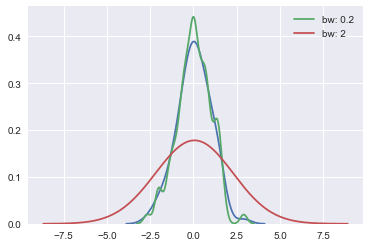y = np.random.randn(100)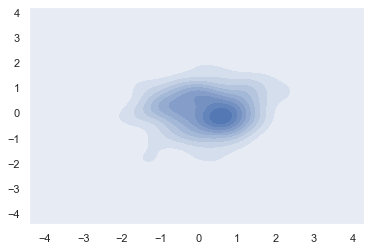cbar：参数若为True，则会添加一个颜色棒(颜色帮在二元kde图像中才有)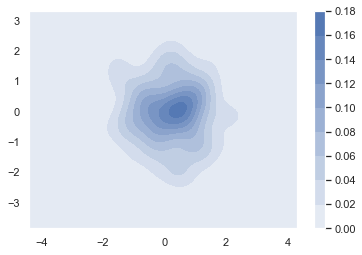sns.distplot(x,color="g")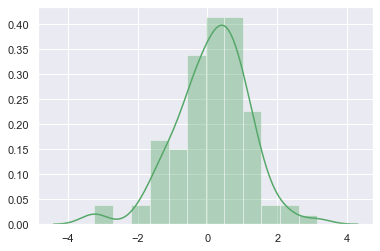import matplotlib.pyplot as plt
fig,axes = plt.subplots(1,3) #创建一个一行三列的画布
sns.distplot(x,ax=axes) #左图
sns.distplot(x,hist=False,ax=axes) #中图
sns.distplot(x,kde=False,ax=axes) #右图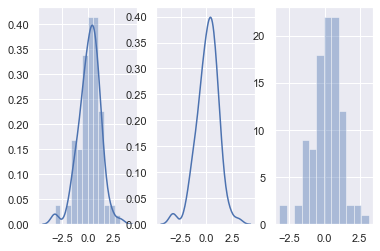bins：int或list，控制直方图的划分

fig,axes=plt.subplots(1,2)
sns.distplot(x,kde=False,bins=20,ax=axes) #左图：分成20个区间
sns.distplot(x,kde=False,bins=[x for x in range(4)],ax=axes) #右图：以0,1,2,3为分割点，形成区间[0,1],[1,2],[2,3]，区间外的值不计入。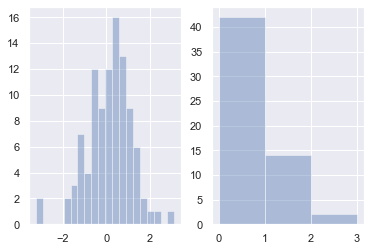rag：控制是否生成观测数值的小细条

fig,axes=plt.subplots(1,2)
sns.distplot(x,rug=True,ax=axes) #左图
sns.distplot(x,ax=axes) #右图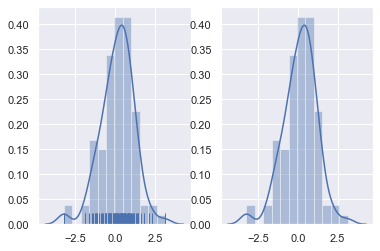fit：控制拟合的参数分布图形，能够直观地评估它与观察数据的对应关系(黑色线条为确定的分布)

from scipy.stats import norm
sns.distplot(x,hist=False,fit=norm) #拟合标准正态分布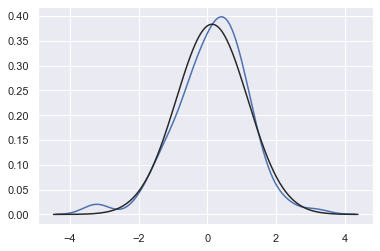from scipy.stats import gamma
z = np.random.gamma(6, size=200)
sns.distplot(z, kde=False, fit=gamma);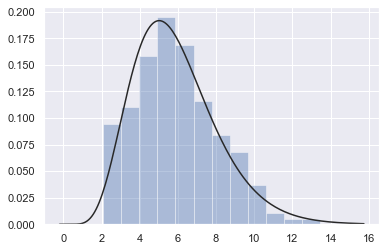hist_kws, kde_kws, rug_kws, fit_kws参数接收字典类型，可以自行定义更多高级的样式

sns.distplot(x,kde_kws={"label":"KDE"},vertical=True,color="y")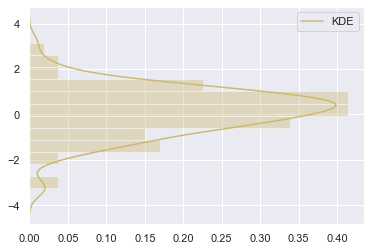norm_hist：若为True, 则直方图高度显示密度而非计数(含有kde图像中默认为True)

fig,axes=plt.subplots(1,2)
sns.distplot(x,norm_hist=True,kde=False,ax=axes) #左图
sns.distplot(x,kde=False,ax=axes) #右图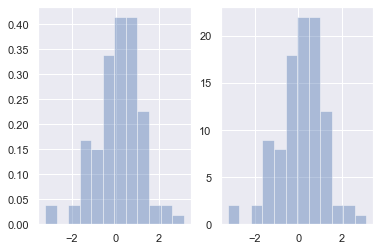sns.jointplot(x,y);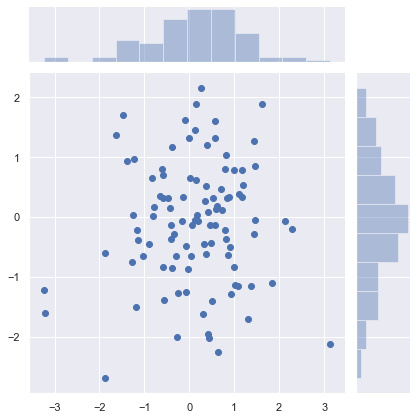sns.jointplot(x, y, kind="hex", color="k")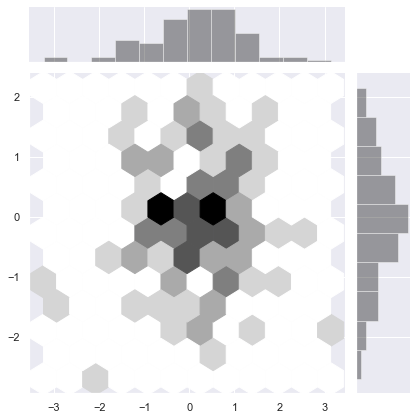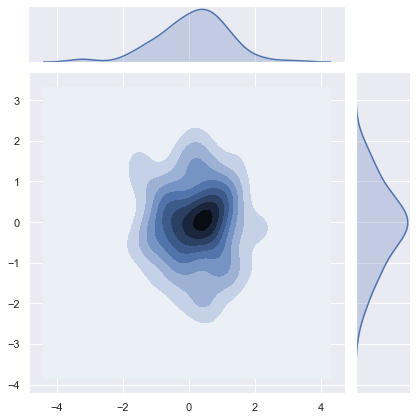f, ax = plt.subplots(figsize=(6, 6))
sns.kdeplot(x, y, ax=ax)
sns.rugplot(x, color="g", ax=ax)
sns.rugplot(y, vertical=True, ax=ax);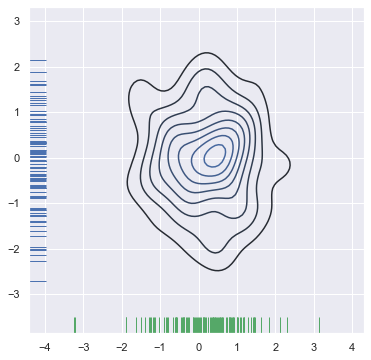jointplot()函数使用JointGrid来管理。为了获得更多的灵活性，您可能需要直接使用JointGrid绘制图形。jointplot()在绘制后返回JointGrid对象，您可以使用它来添加更多图层或调整可视化的其他方面：

g = sns.jointplot(x, y, kind="kde", color="m")
g.plot_joint(plt.scatter, c="w", s=30, linewidth=1, marker="+")
g.ax_joint.collections.set_alpha(0)
g.set_axis_labels("$X$", "$Y$");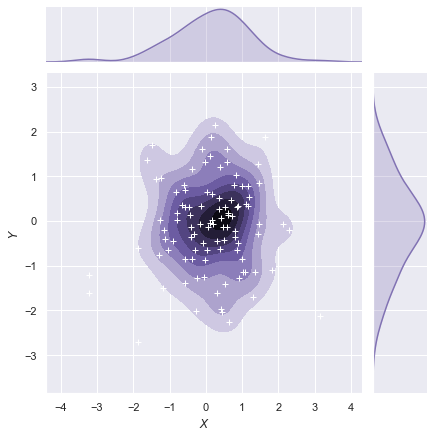sns.pairplot(iris);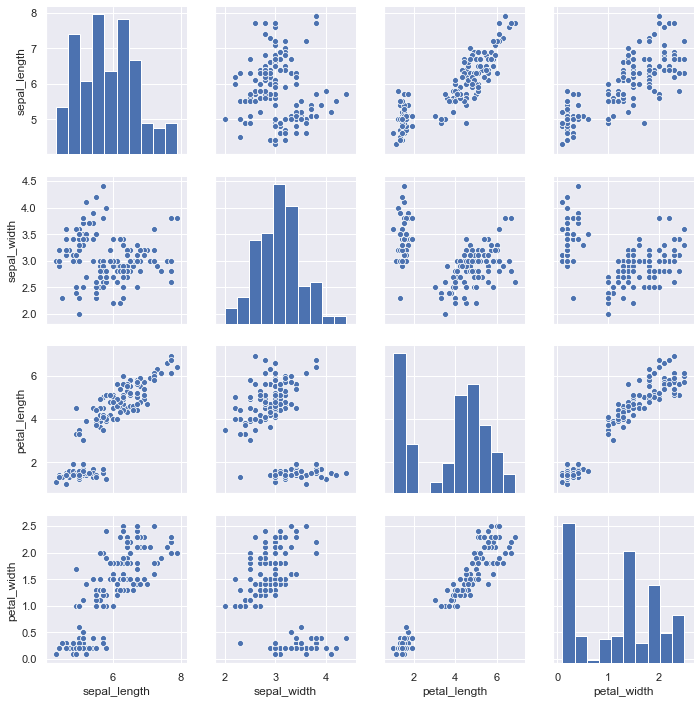g = sns.PairGrid(iris)
g.map_diag(sns.kdeplot)
g.map_offdiag(sns.kdeplot, cmap="Blues_d", n_levels=6);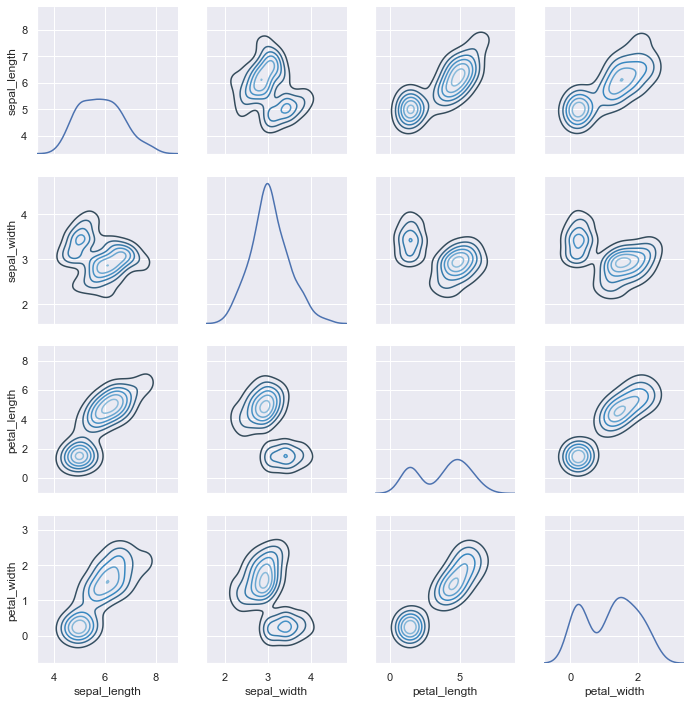sns.pairplot(iris, hue='species', size=3)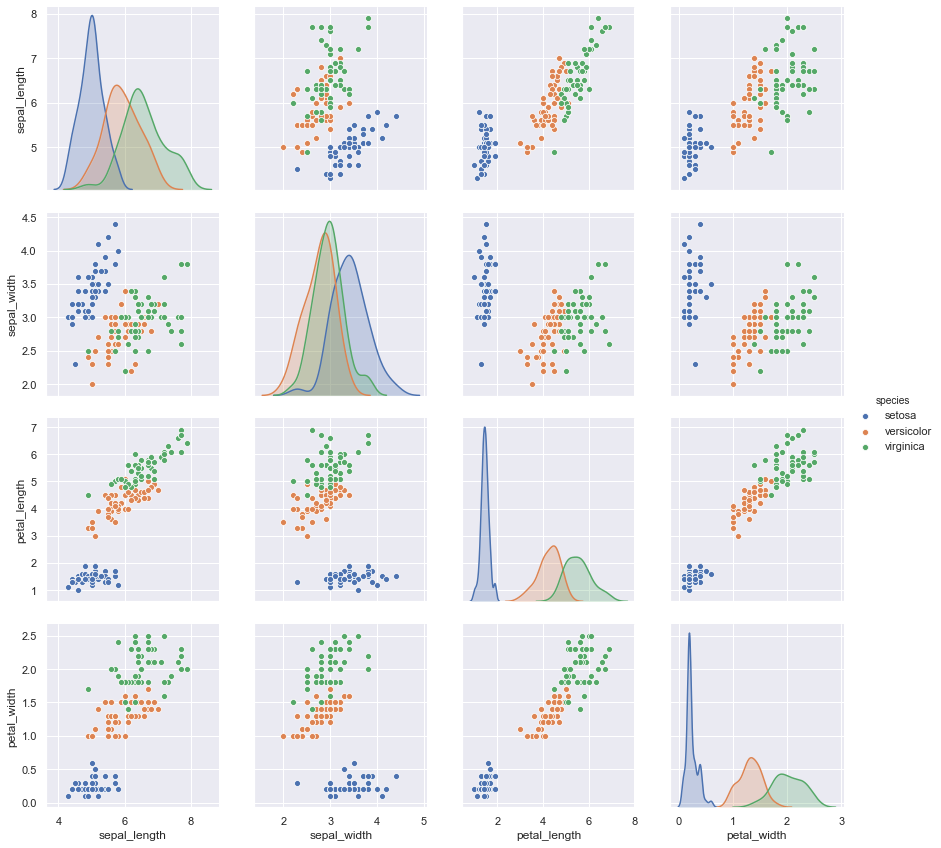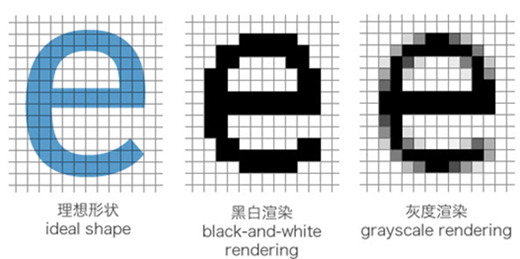网站与APP开发中的字体设置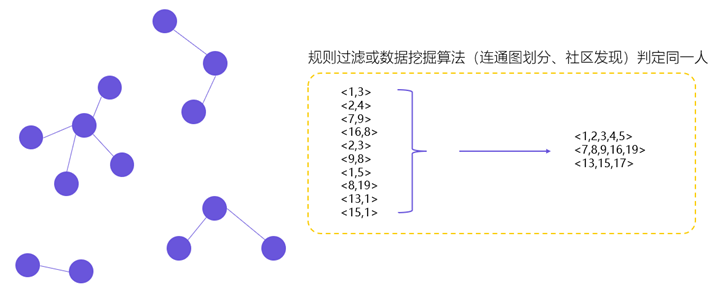用户体系搭建之ID-Mapping